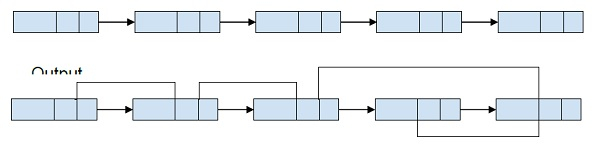# Point arbit pointer to greatest value right side node in a linked list in C++

C++Server Side ProgrammingProgramming

In this problem, we are given a linked list with a value, link pointer and an arbitrary pointer. Our task is to make the arbitrary pointer point to point the largest value which is on the right side of it in the linked list.

Let’s take an example to understand the problem,Here, we can see the following arbitrary pointers of the linked list, which points to the greatest elements on the right side of the linked list.

12 -> 76, 76 -> 54, 54 -> 8, 8 -> 41

To solve this problem, we need to find the greatest element to the right of a node. For this we will do traversal of the linked list in a reverse direction and start finding all the greatest elements and then at each node be will make the arbitrary point to the largest node that we are maintaining.

## Example

Program to show the implementation of our solution,

Live Demo

#include<bits/stdc++.h>
using namespace std;
struct Node{
int data;
Node* next, *arbitrary;
};
Node *prev = NULL, *current = head, *next;
while (current != NULL){
next = current->next;
current->next = prev;
prev = current;
current = next;
}
return prev;
}
while (temp != NULL){
temp->arbitrary = max;
if (max->data < temp->data)
max = temp;
temp = temp->next;
}
}
Node *insertNode(int data) {
Node *new_node = new Node;
new_node->data = data;
new_node->next = NULL;
return new_node;
}
int main() {
printf("Linked List with Arbitrary Pointer: \n");
else
cout<<"NULL";
else
cout<<"NULL";
cout << endl;
}
return 0;
}

## Output

Linked List with Arbitrary Pointer:
12->76: 12->76
76->54: 76->54
54->8: 54->41
8->41: 8->41
41->NULL: 41->NULL